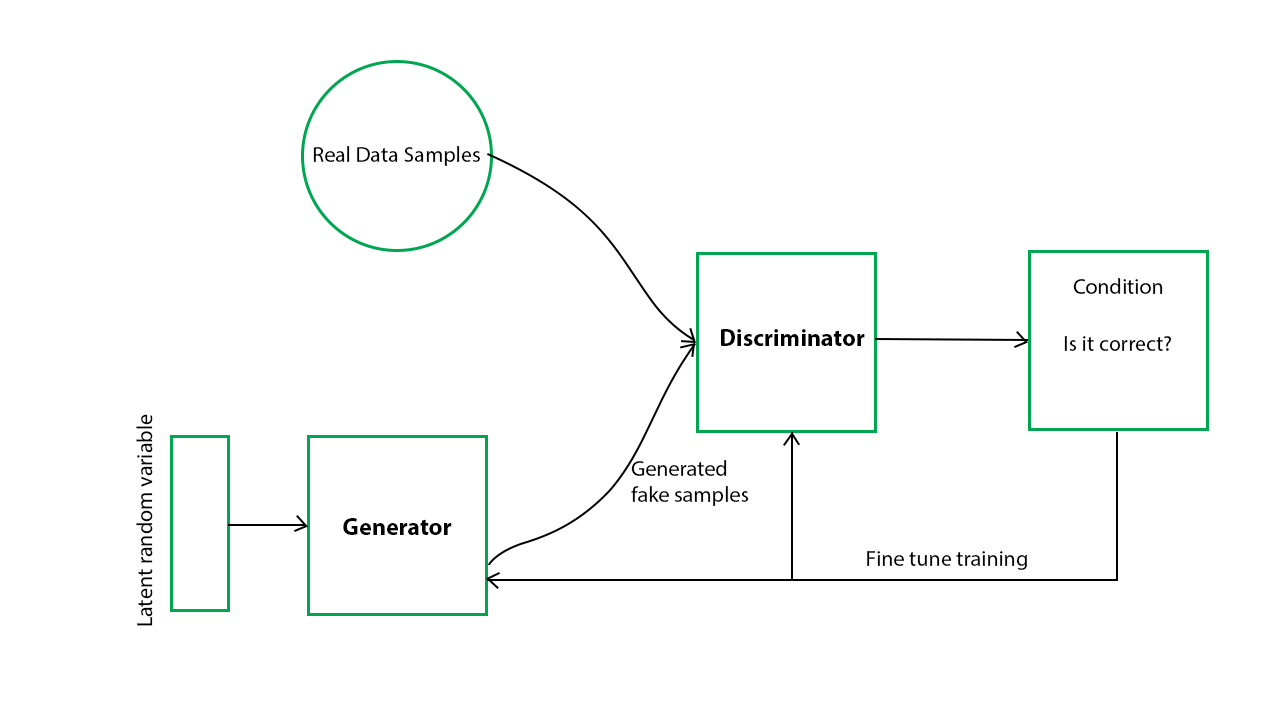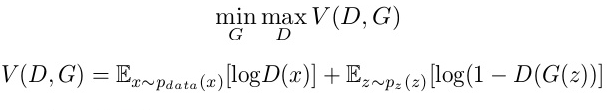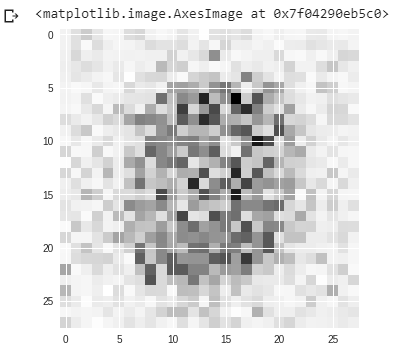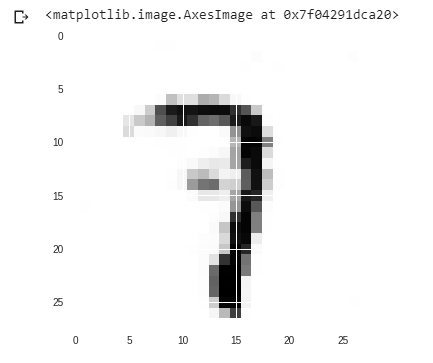• Difficulty Level : Medium
• Last Updated : 15 Jan, 2019

Generative Adversarial Networks (GANs) are a powerful class of neural networks that are used for unsupervised learning. It was developed and introduced by Ian J. Goodfellow in 2014. GANs are basically made up of a system of two competing neural network models which compete with each other and are able to analyze, capture and copy the variations within a dataset.

Why were GANs developed in the first place?
It has been noticed most of the mainstream neural nets can be easily fooled into misclassifying things by adding only a small amount of noise into the original data. Surprisingly, the model after adding noise has higher confidence in the wrong prediction than when it predicted correctly. The reason for such adversary is that most machine learning models learn from a limited amount of data, which is a huge drawback, as it is prone to overfitting. Also, the mapping between the input and the output is almost linear. Although, it may seem that the boundaries of separation between the various classes are linear, but in reality, they are composed of linearities and even a small change in a point in the feature space might lead to misclassification of data.

How does GANs work?

Generative Adversarial Networks (GANs) can be broken down into three parts:

• Generative: To learn a generative model, which describes how data is generated in terms of a probabilistic model.
• Adversarial: The training of a model is done in an adversarial setting.
• Networks: Use deep neural networks as the artificial intelligence (AI) algorithms for training purpose.

In GANs, there is a generator and a discriminator. The Generator generates fake samples of data(be it an image, audio, etc.) and tries to fool the Discriminator. The Discriminator, on the other hand, tries to distinguish between the real and fake samples. The Generator and the Discriminator are both Neural Networks and they both run in competition with each other in the training phase. The steps are repeated several times and in this, the Generator and Discriminator get better and better in their respective jobs after each repetition. The working can be visualized by the diagram given below:Here, the generative model captures the distribution of data and is trained in such a manner that it tries to maximize the probability of the Discriminator in making a mistake. The Discriminator, on the other hand, is based on a model that estimates the probability that the sample that it got is received from the training data and not from the Generator.
The GANs are formulated as a minimax game, where the Discriminator is trying to minimize its reward V(D, G) and the Generator is trying to minimize the Discriminator’s reward or in other words, maximize its loss. It can be mathematically described by the formula below:where,
G = Generator
D = Discriminator
Pdata(x) = distribution of real data
P(z) = distribution of generator
x = sample from Pdata(x)
z = sample from P(z)
D(x) = Discriminator network
G(z) = Generator network

So, basically, training a GAN has two parts:

• Part 1: The Discriminator is trained while the Generator is idle. In this phase, the network is only forward propagated and no back-propagation is done. The Discriminator is trained on real data for n epochs, and see if it can correctly predict them as real. Also, in this phase, the Discriminator is also trained on the fake generated data from the Generator and see if it can correctly predict them as fake.
• Part 2: The Generator is trained while the Discriminator is idle. After the Discriminator is trained by the generated fake data of the Generator, we can get its predictions and use the results for training the Generator and get better from the previous state to try and fool the Discriminator.
• The above method is repeated for a few epochs and then manually check the fake data if it seems genuine. If it seems acceptable, then the training is stopped, otherwise, its allowed to continue for few more epochs.

Different types of GANs:
GANs are now a very active topic of research and there have been many different types of GAN implementation. Some of the important ones that are actively being used currently are described below:

1. Vanilla GAN: This is the simplest type GAN. Here, the Generator and the Discriminator are simple multi-layer perceptrons. In vanilla GAN, the algorithm is really simple, it tries to optimize the mathematical equation using stochastic gradient descent.
2. Conditional GAN (CGAN): CGAN can be described as a deep learning method in which some conditional parameters are put into place. In CGAN, an additional parameter ‘y’ is added to the Generator for generating the corresponding data. Labels are also put into the input to the Discriminator in order for the Discriminator to help distinguish the real data from the fake generated data.
3. Deep Convolutional GAN (DCGAN): DCGAN is one of the most popular also the most successful implementation of GAN. It is composed of ConvNets in place of multi-layer perceptrons. The ConvNets are implemented without max pooling, which is in fact replaced by convolutional stride. Also, the layers are not fully connected.
4. Laplacian Pyramid GAN (LAPGAN): The Laplacian pyramid is a linear invertible image representation consisting of a set of band-pass images, spaced an octave apart, plus a low-frequency residual. This approach uses multiple numbers of Generator and Discriminator networks and different levels of the Laplacian Pyramid. This approach is mainly used because it produces very high-quality images. The image is down-sampled at first at each layer of the pyramid and then it is again up-scaled at each layer in a backward pass where the image acquires some noise from the Conditional GAN at these layers until it reaches its original size.
5. Super Resolution GAN (SRGAN): SRGAN as the name suggests is a way of designing a GAN in which a deep neural network is used along with an adversarial network in order to produce higher resolution images. This type of GAN is particularly useful in optimally up-scaling native low-resolution images to enhance its details minimizing errors while doing so.

Sample Python code implementing a Generative Adversarial Network:
GANs are very computationally expensive. They require high powered GPUs and a lot of time (a large number of epochs) to produce good results. For our example, we will be using the famous MNIST dataset and use it to produce a clone of a random digit.

 `# importing the necessary libraries and the MNIST dataset``import` `tensorflow as tf``import` `numpy as np``import` `matplotlib.pyplot as plt``from` `tensorflow.examples.tutorials.mnist ``import` `input_data`` ` `mnist ``=` `input_data.read_data_sets(``"MNIST_data"``)`` ` `# defining functions for the two networks.``# Both the networks have two hidden layers``# and an output layer which are densely or ``# fully connected layers defining the ``# Generator network function``def` `generator(z, reuse ``=` `None``):``    ``with tf.variable_scope(``'gen'``, reuse ``=` `reuse):``        ``hidden1 ``=` `tf.layers.dense(inputs ``=` `z, units ``=` `128``, ``                            ``activation ``=` `tf.nn.leaky_relu)``                             ` `        ``hidden2 ``=` `tf.layers.dense(inputs ``=` `hidden1,``           ``units ``=` `128``, activation ``=` `tf.nn.leaky_relu)``            ` `        ``output ``=` `tf.layers.dense(inputs ``=` `hidden2, ``             ``units ``=` `784``, activation ``=` `tf.nn.tanh)``         ` `        ``return` `output`` ` `# defining the Discriminator network function ``def` `discriminator(X, reuse ``=` `None``):``    ``with tf.variable_scope(``'dis'``, reuse ``=` `reuse):``        ``hidden1 ``=` `tf.layers.dense(inputs ``=` `X, units ``=` `128``,``                            ``activation ``=` `tf.nn.leaky_relu)``                             ` `        ``hidden2 ``=` `tf.layers.dense(inputs ``=` `hidden1,``               ``units ``=` `128``, activation ``=` `tf.nn.leaky_relu)``                ` `        ``logits ``=` `tf.layers.dense(hidden2, units ``=` `1``)``        ``output ``=` `tf.sigmoid(logits)``         ` `        ``return` `output, logits`` ` `# creating placeholders for the outputs``tf.reset_default_graph()`` ` `real_images ``=` `tf.placeholder(tf.float32, shape ``=``[``None``, ``784``])``z ``=` `tf.placeholder(tf.float32, shape ``=``[``None``, ``100``])`` ` `G ``=` `generator(z)``D_output_real, D_logits_real ``=` `discriminator(real_images)``D_output_fake, D_logits_fake ``=` `discriminator(G, reuse ``=` `True``)`` ` `# defining the loss function``def` `loss_func(logits_in, labels_in):``    ``return` `tf.reduce_mean(tf.nn.sigmoid_cross_entropy_with_logits(``                          ``logits ``=` `logits_in, labels ``=` `labels_in))`` ` ` ``# Smoothing for generalization``D_real_loss ``=` `loss_func(D_logits_real, tf.ones_like(D_logits_real)``*``0.9``)``D_fake_loss ``=` `loss_func(D_logits_fake, tf.zeros_like(D_logits_real))``D_loss ``=` `D_real_loss ``+` `D_fake_loss`` ` `G_loss ``=` `loss_func(D_logits_fake, tf.ones_like(D_logits_fake))`` ` `# defining the learning rate, batch size,``# number of epochs and using the Adam optimizer``lr ``=` `0.001` `# learning rate`` ` `# Do this when multiple networks``# interact with each other`` ` `# returns all variables created(the two``# variable scopes) and makes trainable true``tvars ``=` `tf.trainable_variables() ``d_vars ``=``[var ``for` `var ``in` `tvars ``if` `'dis'` `in` `var.name]``g_vars ``=``[var ``for` `var ``in` `tvars ``if` `'gen'` `in` `var.name]`` ` `D_trainer ``=` `tf.train.AdamOptimizer(lr).minimize(D_loss, var_list ``=` `d_vars)``G_trainer ``=` `tf.train.AdamOptimizer(lr).minimize(G_loss, var_list ``=` `g_vars)`` ` `batch_size ``=` `100` `# batch size``epochs ``=` `500` `# number of epochs. The higher the better the result``init ``=` `tf.global_variables_initializer()`` ` `# creating a session to train the networks``samples ``=``[] ``# generator examples`` ` `with tf.Session() as sess:``    ``sess.run(init)``    ``for` `epoch ``in` `range``(epochs):``        ``num_batches ``=` `mnist.train.num_examples``/``/``batch_size``         ` `        ``for` `i ``in` `range``(num_batches):``            ``batch ``=` `mnist.train.next_batch(batch_size)``            ``batch_images ``=` `batch[``0``].reshape((batch_size, ``784``))``            ``batch_images ``=` `batch_images ``*` `2``-``1``            ``batch_z ``=` `np.random.uniform(``-``1``, ``1``, size ``=``(batch_size, ``100``))``            ``_``=` `sess.run(D_trainer, feed_dict ``=``{real_images:batch_images, z:batch_z})``            ``_``=` `sess.run(G_trainer, feed_dict ``=``{z:batch_z})``             ` `        ``print``(``"on epoch{}"``.``format``(epoch))``         ` `        ``sample_z ``=` `np.random.uniform(``-``1``, ``1``, size ``=``(``1``, ``100``))``        ``gen_sample ``=` `sess.run(generator(z, reuse ``=` `True``),``                                 ``feed_dict ``=``{z:sample_z})``         ` `        ``samples.append(gen_sample)`` ` `# result after 0th epoch``plt.imshow(samples[``0``].reshape(``28``, ``28``))`` ` `# result after 499th epoch``plt.imshow(samples[``49``].reshape(``28``, ``28``))`

#### Output:

```on epoch0
on epoch1
...
...
...
on epoch498
on epoch499
```

Result after 0th epoch:Resulr after 499th epoch:So, from the above example, we see that in the first image after 0th epoch, the pixels are scattered all over the place and we couldn’t figure anything out from it.
But from the second image, we could see that the pixels are more systematically organized and we could figure out that it is the digit ‘7’ that the code has randomly chosen and the network has tried to make a clone of it. In our example, we have taken 500 as the number of epochs. But you could increase that number to further refine your result.

My Personal Notes arrow_drop_up